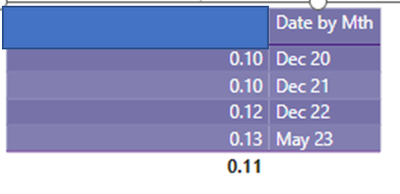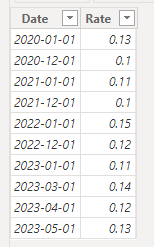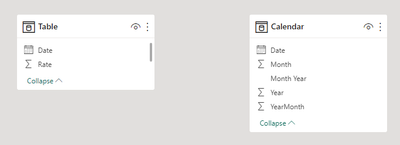cancel
Showing results for
Did you mean:Helper II

## DAX use value of the month as value for December

Hello everyone,

I have a pourcentage that display the ratio at the last period available (May), I need to use this value as value for end of the year (december)

I create a measure using dax to replace the blank by the value I want but it do not returns me the value for decemberHow can I have the value of May 23 as Dec 23,

Below the dax code, thank you for your support

``````%  Evolution  =

VAR Closing = LASTDATE(ALL('DimDate Closing Period'[Date]))

VAR Max_Period = YEAR(LASTDATE(ALL('DimDate Closing Period'[Date])))+2

VAR Current_Year = YEAR(LASTDATE(ALL('DimDate Closing Period'[Date])))

VAR End_Year =

CALCULATE( [% Female M & Above],
FILTER (
'DimDate',
MONTH ( 'DimDate'[Date]) = 12
&& YEAR ( 'DimDate'[Date] ) >= Current_Year - 3 && YEAR('DimDate'[Date]) <= Max_Period)
)

VAR Last_Value =

CALCULATE(
[% Value],
FILTER ('DimDate Closing Period',
'DimDate Closing Period'[Date] = Closing)
)

RETURN

SWITCH(TRUE(),
NOT ISBLANK(End_Year), End_Year,
ISBLANK(End_Year), last_Value,
BLANK()
)``````

1 ACCEPTED SOLUTIONCommunity Support

Hi @Fantmas ,

It seems that you want to show the last rate in each year as Dec month value. I suggest you to create a dimdate table with continuous date to help calculation.

Sample:Measure:

``````Measure =
VAR _LastDateEachYear =
CALCULATE (
MAX ( 'Table'[Date] ),
FILTER (
ALL ( 'Table' ),
YEAR ( 'Table'[Date] ) = YEAR ( MAX ( 'Calendar'[Date] ) )
)
)
VAR _RATE =
CALCULATE (
SUM ( 'Table'[Rate] ),
FILTER (
ALL ( 'Table' ),
YEAR ( 'Table'[Date] ) = MAX ( 'Calendar'[Year] )
&& 'Table'[Date] = _LastDateEachYear
)
)
RETURN
IF ( MAX ( 'Calendar'[Month] ) = 12, _RATE )``````

Result is as below.Best Regards,
Rico Zhou

If this post helps, then please consider Accept it as the solution to help the other members find it more quickly.

2 REPLIES 2Community Support

Hi @Fantmas ,

It seems that you want to show the last rate in each year as Dec month value. I suggest you to create a dimdate table with continuous date to help calculation.

Sample:Measure:

``````Measure =
VAR _LastDateEachYear =
CALCULATE (
MAX ( 'Table'[Date] ),
FILTER (
ALL ( 'Table' ),
YEAR ( 'Table'[Date] ) = YEAR ( MAX ( 'Calendar'[Date] ) )
)
)
VAR _RATE =
CALCULATE (
SUM ( 'Table'[Rate] ),
FILTER (
ALL ( 'Table' ),
YEAR ( 'Table'[Date] ) = MAX ( 'Calendar'[Year] )
&& 'Table'[Date] = _LastDateEachYear
)
)
RETURN
IF ( MAX ( 'Calendar'[Month] ) = 12, _RATE )``````

Result is as below.Best Regards,
Rico Zhou

If this post helps, then please consider Accept it as the solution to help the other members find it more quickly.Super User

Please provide sample data (with sensitive information removed) that covers your issue or question completely, in a usable format (not as a screenshot).
https://community.fabric.microsoft.com/t5/Community-Blog/How-to-provide-sample-data-in-the-Power-BI-...
Please show the expected outcome based on the sample data you provided.Announcements#### Exclusive opportunity for Women!

Join us for a free, hands-on Microsoft workshop led by women trainers for women where you will learn how to build a Dashboard in a Day!#### Power Platform Conference-Power BI and Fabric Sessions

Join us Oct 1 - 6 in Las Vegas for the Microsoft Power Platform Conference.Top Solution Authors
Top Kudoed Authors
Users online (1,711)